## Monday, March 5, 2018

### Some new functions for teaching phenotypic evolution

For the Evolution class I'm teaching this semester I've been working on adding some basic population genetic functions to my GitHub R package PopGen.

Today, the first one is a function to compute Hardy-Weinberg frequencies, but for an arbitrary number of alleles.

First, here is what the function looks like:

``````hardy.weinberg<-function(p=c(0.5,0.5),alleles=c("A","a"),as.matrix=FALSE){
if(sum(p)!=1) p<-p/sum(p)
nalleles<-max(length(p),length(alleles))
if(nalleles!=length(p)) p<-rep(1/nalleles,nalleles)
if(nalleles!=length(alleles)) {
if(length(p)<=26) alleles<-LETTERS[1:length(p)]
else alleles<-paste("A",1:length(p),sep="")
}
p<-setNames(p,alleles)
HW<-as.matrix(p)%*%t(as.matrix(p))
if(as.matrix) return(HW)
else {
hw<-vector()
k<-1
for(i in 1:length(alleles)){
for(j in i:length(alleles)){
hw[k]<-if(i==j) HW[i,j] else 2*HW[i,j]
names(hw)[k]<-paste(alleles[c(i,j)],collapse="")
k<-k+1
}
}
return(hw)
}
}
``````

Now let's use it. Obviously, it is straightforward in the biallelic case:

``````hardy.weinberg(p=c(0.7,0.3))
``````
``````##   AA   Aa   aa
## 0.49 0.42 0.09
``````
``````## or
barplot(hardy.weinberg(p=c(0.7,0.3)))
``````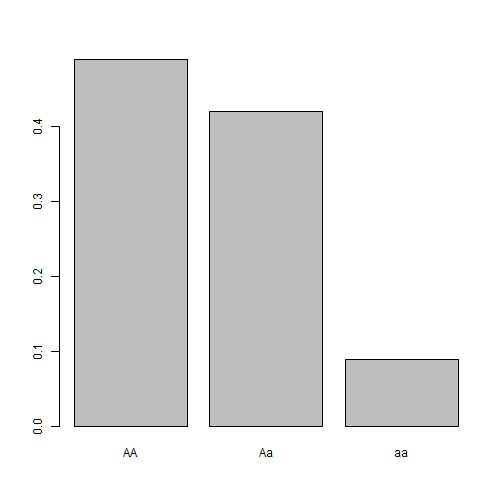but it also works nicely for multi-allelic situations in which Hardy-Weinberg frequencies are more laborious to compute:

``````hw<-hardy.weinberg(p=c(0.4,0.3,0.2,0.1))
hw
``````
``````##   AA   AB   AC   AD   BB   BC   BD   CC   CD   DD
## 0.16 0.24 0.16 0.08 0.09 0.12 0.06 0.04 0.04 0.01
``````
``````barplot(hw)
``````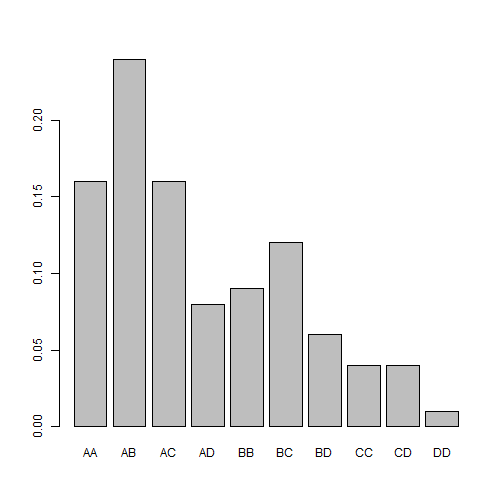The second function is designed merely to show that for multilocus genotypes in which allelic substitutions have additive effect, we quickly converge on a (approximately) normal phenotypic distribution for even a modest number of loci. Furthermore, that this is true regardless of our allele frequencies.

The way the function works is by computing all multi-locus genotypes and their HW frequencies, and then plotting the resultant phenotypic trait distribution.

Here's the function:

``````phenotype.freq<-function(nloci=10,p=NULL,effect=1){
if(is.null(p)) p<-rep(0.5,nloci)
genotypes<-t(apply(cbind(p,1-p),1,hardy.weinberg))
COMBN<-permutations(n=3,r=nloci,set=T,repeats.allowed=T)
PHEN<-rowSums(COMBN-2)*effect
FREQ<-rep(1,nrow(COMBN))
for(i in 1:nrow(COMBN)){
for(j in 1:nloci)   FREQ[i]<-FREQ[i]*genotypes[j,COMBN[i,j]]
}
phen<-unique(PHEN)
freq<-rep(0,length(phen))
for(i in 1:length(phen)) freq[i]<-sum(FREQ[which(PHEN==phen[i])])
plot(phen,freq,type="b",pch=21,bg="grey",
xlab="phenotypic trait value",ylab="relative frequency",
cex=1.5,xlim=range(phen)+c(-0.5,0.5)*effect)
for(i in 1:length(phen))
rect(phen[i]-0.4*effect,0,phen[i]+0.4*effect,
freq[i],border="grey",
col=make.transparent("blue",0.2))
}
``````

and here's what I mean using random allele frequencies at each locus (note that this function uses gtools & phytools):

``````library(gtools)
library(phytools)
## 1 locus
nloci<-1
phenotype.freq(nloci,p=runif(n=nloci),effect=1/nloci)
``````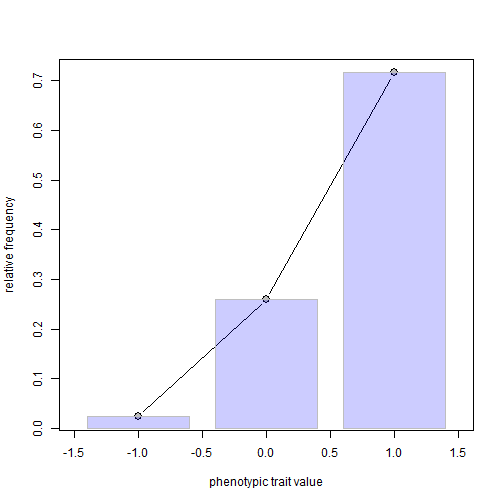``````## 2 loci
nloci<-2
phenotype.freq(nloci,p=runif(n=nloci),effect=1/nloci)
``````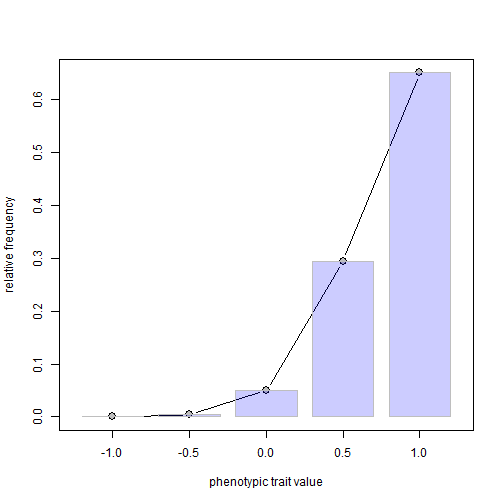``````## 4 loci
nloci<-4
phenotype.freq(nloci,p=runif(n=nloci),effect=1/nloci)
``````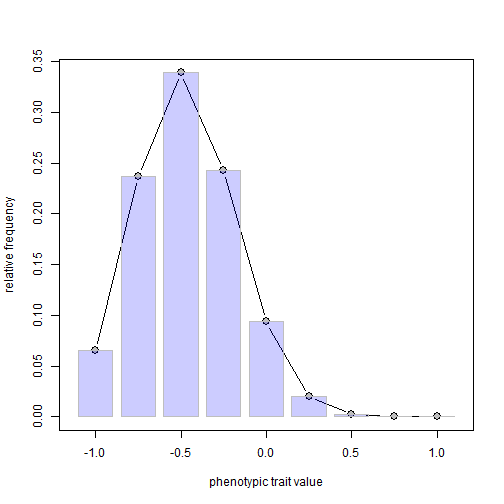``````## 8 loci
nloci<-8
phenotype.freq(nloci,p=runif(n=nloci),effect=1/nloci)
``````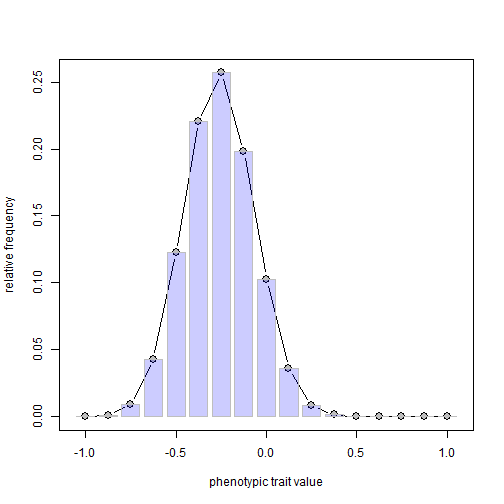``````## 12 loci
nloci<-12
phenotype.freq(nloci,p=runif(n=nloci),effect=1/nloci)
``````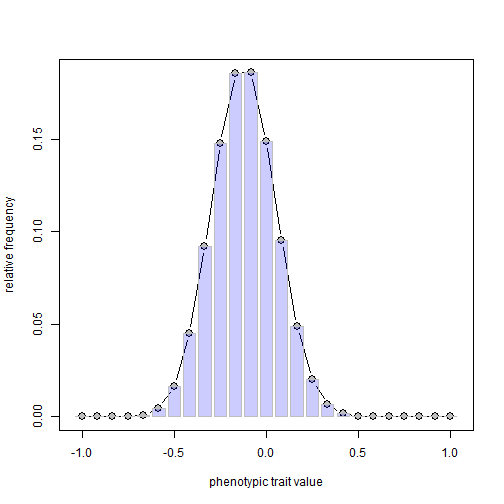Neat? I kind of think so.

Note that because the function finds all possible multilocus genotypes for `nloci` greater than 12 or 14 it is not going to work!